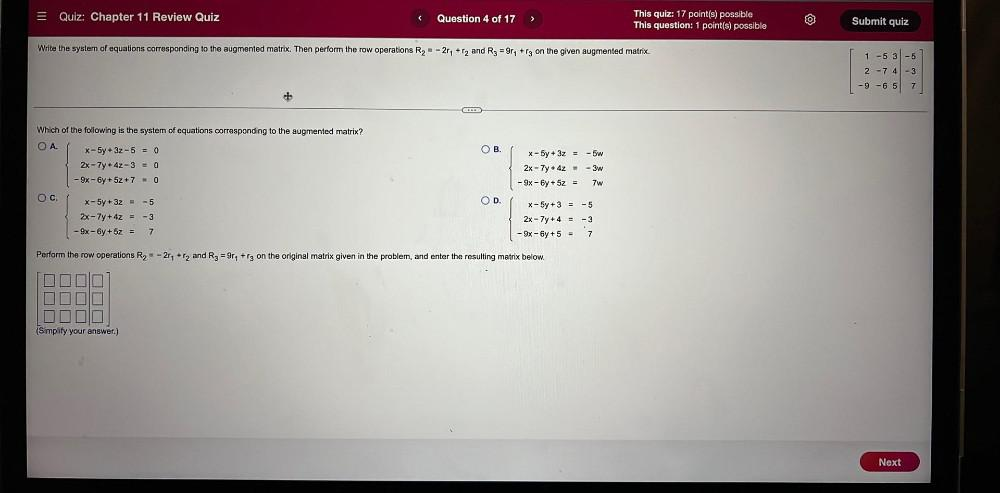Question:

# = Quiz: Chapter 11 Review Quiz Question 4 of 17 > This quiz: 17 point(s) possible This question: 1 point(s) possible Submit quiz= Quiz: Chapter 11 Review Quiz Question 4 of 17 > This quiz: 17 point(s) possible This question: 1 point(s) possible Submit quiz Write the system of equations corresponding to the augmented matrix. Then perform the row operations R2 = -217 +r2 and Rg = 9r+rg on the given augmented matrix. 1 -5 3-5 2 - 74 -3 -9-65 7 + Which of the following is the system of equations corresponding to the augmented matrix? O A. OB. - 5w X-5y + 3z-5 = 0 2x - 7y + 4z - 3 = 0 - 9x - 6y + 5z + 7 = 0 X-5y + 3z = 2x - 7y + 4z = - 9x - 6y + 5z = -3w 7w O c. OD -5 X-5y + 3z = -5 2x - 7y + 4z = -3 - 9x - 6y + 5z = 7 x - 5y + 3 = 2x - 7y +4 = - 9x - 6y + 5 = -3 7 Perform the row operations R2 = -277 + r2 and R3 =914 + rg on the original matrix given in the problem, and enter the resulting matrix below. LL (Simplify your answer.) Next